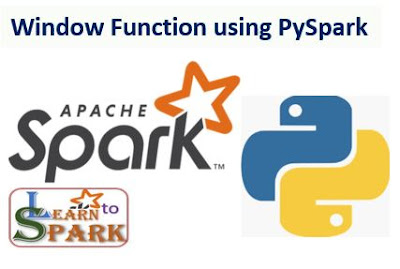# How to Select First Row of Each Group in Spark | Window Function using PySpark | Apache Spark TutorialIn this post, we will learn about window function and discuss in detail about the types and how to code in PySpark to achieve the concept of windowing function. As usual, we will take some samples and do hands-on with it to understand the entire concept clearly. Come, lets get started with today's article.

### Problem Statement:

For today's problem statement, consider we have a data of items sold per month along with price and type of merchant in a CSV file. The sample input data is shown in the below figure.

We need to,

• Find the top-selling product in each type and order them by the revenue.

### What is Window Function:

Window Function, was introduced with the SparkSql from Spark version 1.4 and on an overview, it is a Function that offers the user of Spark with an extended capability to  perform the wide range of operation such as calculating the moving average of the given input range of rows, max value, min value, least value etc., over any given range of records. It allows the developer to calculate the value for each input record based on the range of records in consideration and returns a single value for each and every input record. Every input records can have their own group of records associated with it for processing. This group or range of records are called frames. Window function is one of the most powerful one used by developers to express various operation and data processing that are really hard to manipulate without this function

### How to Use Window Function:

Window Function can be used in both Spark SQL and with Spark Dataframe API. The general syntax to define the window function in PySpark is as follows

Method 1: Using Spark SQL, to define window we should use the supporting Spark Sql function followed by over() clause.

OVER (PARTITION BY ... ORDER BY ...)

eg;  SELECT col1,
col2,
col3,
{Sql function} OVER(PARTITION BY col1 ORDER BY col2) as col4
FROM table name

Method 2; We can use window function on top of Spark Dataframe as well. we need to use same Over() clause as shown in below sample snippet. Three key items to look in our syntax after over() clause are

• Partition Specification
• Ordering Specification
• Frame Specification

over(Window.partitionBy(...).orderBy(...))

eg: df.select(
df['col1'],
df['col2'],
df['col3'],
{func}(df['col3']).over(window.partitionby(df['col1'])).orderby(df['col2']).alias("col4")
)

For now just have a look into syntax, we will later have hands-on using this syntax to solve our problem statement. Without window function, it will be difficult for our developers to calculate the values, as it might include lot of effort to split the data into separate table for each category and finally join all the tables for the consolidated result. Before moving on to our hands-on, let us also learn about the types of functions supported in Windowing using PySpark.

### Types of Window Function:

Spark extends its support with three kind of window function. They are,
• Ranking Function
• Analytics Function
• Aggregate Function

Below chart gives us the detailed knowledge in the functions available under each type.

Now, as we got some basic idea of what window function is; and type of window function available in Spark, lets quickly move onto the hands-on part of today's post.

### Solution to Problem:

To answer the problem statement, Find the top-selling product for each type and sort by revenue, we need to apply rank function to the product of each type based on the revenue generated by those product. Here we use dense_rank() function to achieve this. Let us see both the SparkSQL and PySpark Dataframe API method to solve this problem

Coding snippet for SparkSQL:

Step 1: Read the input csv file as a Dataframe and create a temporary view name tmpview on top of that DF.

Step 2: Write a select statement as shown below,

SELECT  product,
type,
revenue
FROM (
SELECT product,
type,
revenue,
dense_rank() OVER (PARTITION BY type ORDER BY revenue DESC) as rank
FROM tmpview) tmp
WHERE
rank <= 1

Code Snippet using PySpark Dataframe API:

import sys
from pyspark.sql.window import Window
import pyspark.sql.functions as func

windowSpec = Window.partitionBy(df['type']).orderBy(df['revenue'].desc())
dense_rank_revenue=func.dense_rank().over(windowSpec)

df.select(
df['product'],
df['type'],
df['revenue'],

dense_rank_revenue.alias("Rank")).filter("Rank<=1").show()

### Output:

Intermediate output[]:

Final Output[]:

### Full Program:

Hope you enjoyed learning complete information on windowing concept in PySpark. Provide your comments and support my Facebook page by giving a like and share.

Happy Learning!!!